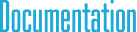#SpatialVector6d Class Reference

## Detailed Description

6D spatial vector (for 3D) with double type elementsInheritance diagram for SpatialVector6d:

## Public Member Functions

Initialization methods
void Set (const Point3< double > &p1, const Point3< double > &p2)

void Set (double a1, double a2, double a3, double b1, double b2, double b3)

void Zero ()

Transpose methods
void SetTranspose ()

SpatialVector6 Transpose () const

Unary operators
SpatialVector6 operator- () const

Binary operators
SpatialVector6 operator- (const SpatialVector6 &s) const

SpatialVector6 operator+ (const SpatialVector6 &s) const

SpatialVector6 operator* (double t) const

double operator* (const SpatialVector6 &s) const

Assignment operators
void operator+= (const SpatialVector6 &s)

void operator-= (const SpatialVector6 &s)

void operator*= (double t)

## Public Attributes

Components
Point3< double > a

Point3< double > b

## § operator-()

 SpatialVector6 operator- ( const SpatialVector6< double > & s ) const
inherited

Scalar product of two vectors. Note that one of the vectors should be motion vector ant the other should be a force vector. Otherwise, scalar product is not defined in spatial vector algebra.

## § operator+()

 SpatialVector6 operator+ ( const SpatialVector6< double > & s ) const
inherited

Scalar product of two vectors. Note that one of the vectors should be motion vector ant the other should be a force vector. Otherwise, scalar product is not defined in spatial vector algebra.

## § operator*() [1/2]

 SpatialVector6 operator* ( double t ) const
inherited

Scalar product of two vectors. Note that one of the vectors should be motion vector ant the other should be a force vector. Otherwise, scalar product is not defined in spatial vector algebra.

## § operator*() [2/2]

 double operator* ( const SpatialVector6< double > & s ) const
inherited

Scalar product of two vectors. Note that one of the vectors should be motion vector ant the other should be a force vector. Otherwise, scalar product is not defined in spatial vector algebra.